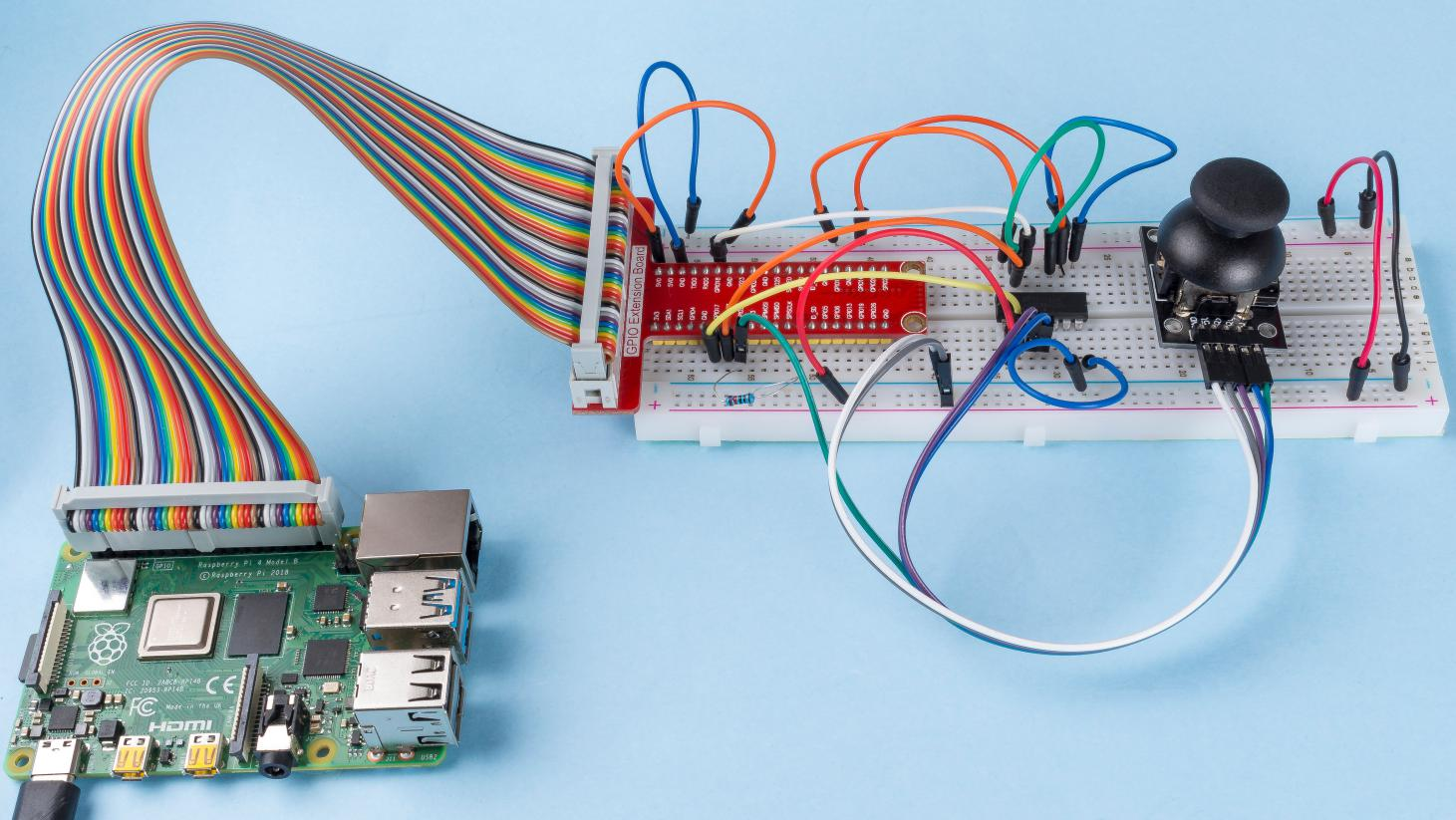# 2.1.9 Joystick¶

## Introduction¶

In this project, We’re going to learn how joystick works. We manipulate the Joystick and display the results on the screen.

## Required Components¶

In this project, we need the following components.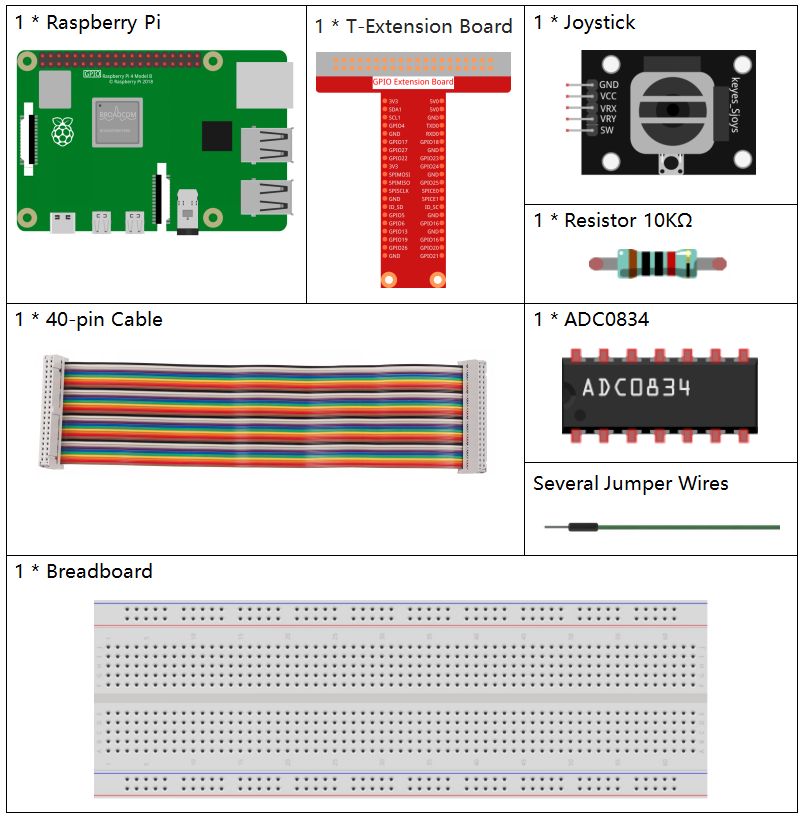Name

ITEMS IN THIS KIT

Raphael Kit

337

Raphael Kit

COMPONENT INTRODUCTION

GPIO Extension Board

Jumper Wires

Resistor

Joystick Module

-

-

## Schematic Diagram¶

When the data of joystick is read, there are some differents between axis: data of X and Y axis is analog, which need to use ADC0834 to convert the analog value to digital value. Data of Z axis is digital, so you can directly use the GPIO to read, or you can also use ADC to read.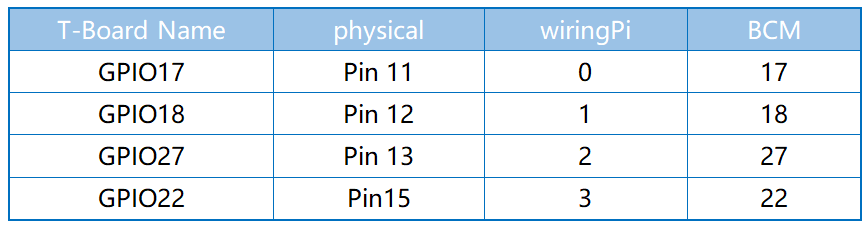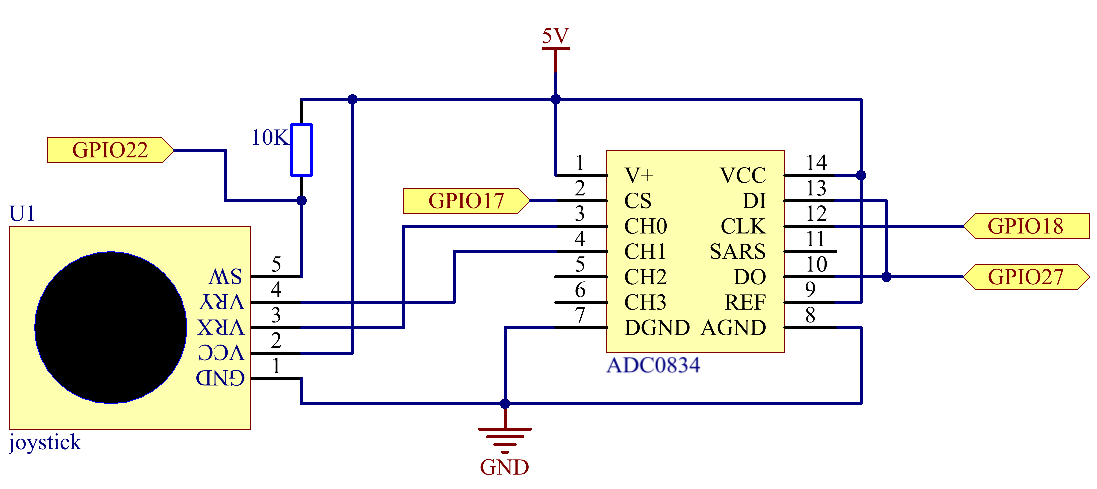## Experimental Procedures¶

Step 1: Build the circuit.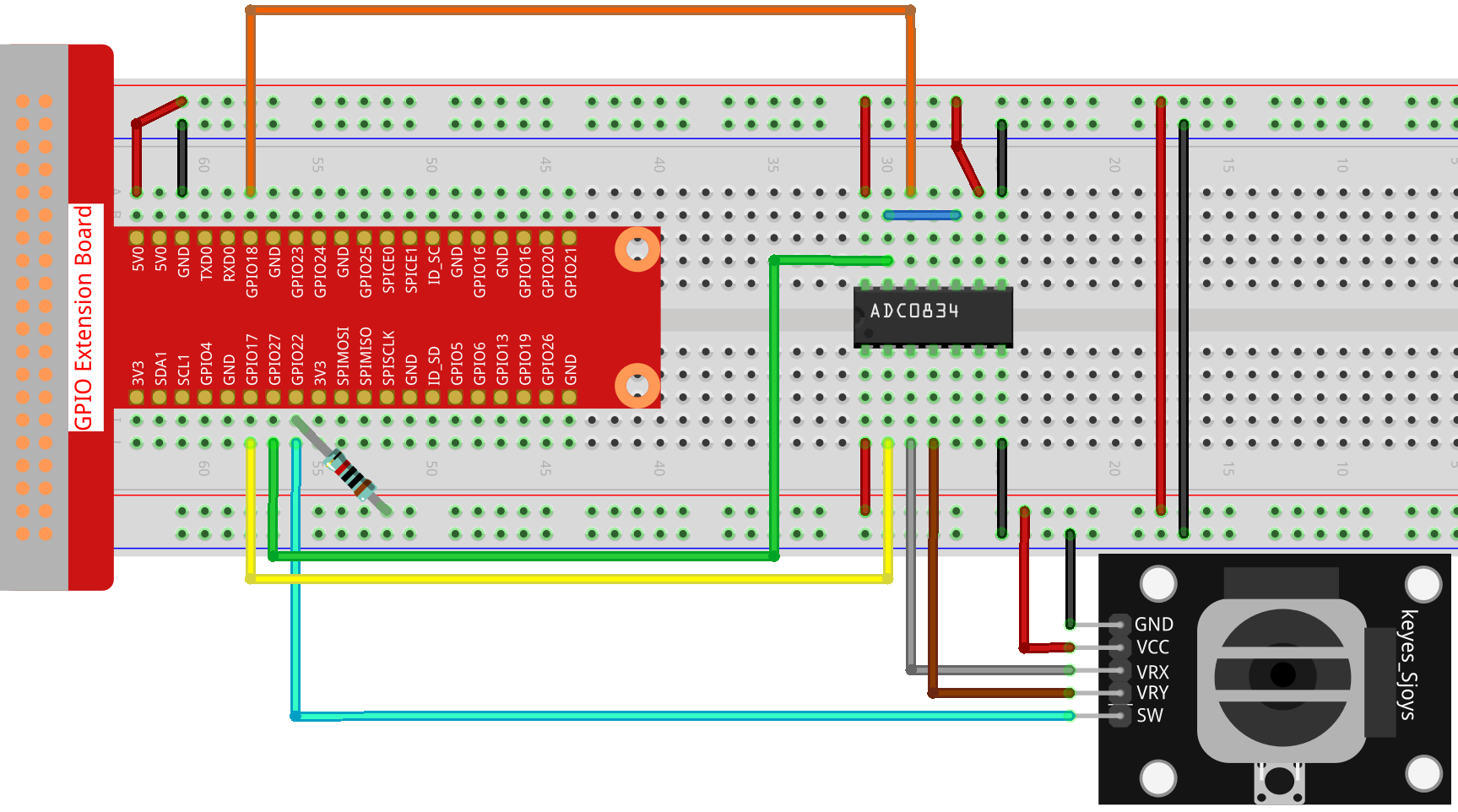Step 2: Go to the folder of the code.

```cd ~/raphael-kit/c/2.1.9/
```

Step 3: Compile the code.

```gcc 2.1.9_Joystick.c -lwiringPi
```

Step 4: Run the executable file.

```sudo ./a.out
```

After the code runs, turn the Joystick, then the corresponding values of x, y, Btn are displayed on screen.

Note

If it does not work after running, or there is an error prompt: "wiringPi.h: No such file or directory", please refer to Install and Check the WiringPi.

Code

```#include <wiringPi.h>
#include <stdio.h>
#include <softPwm.h>

typedef unsigned char uchar;
typedef unsigned int uint;

#define     BtnPin    3

{
uchar i;
uchar dat1=0, dat2=0;
int sel = channel > 1 & 1;
int odd = channel & 1;
// Start bit
//Single End mode
// ODD
//Select
for(i=0;i<8;i++)
{
}
for(i=0;i<8;i++)
{
}
return(dat1==dat2) ? dat1 : 0;
}
int main(void)
{
uchar x_val;
uchar y_val;
uchar btn_val;
if(wiringPiSetup() == -1){ //when initialize wiring failed,print messageto screen
printf("setup wiringPi failed !");
return 1;
}
pinMode(BtnPin,  INPUT);
pullUpDnControl(BtnPin, PUD_UP);

while(1){
printf("x = %d, y = %d, btn = %d\n", x_val, y_val, btn_val);
delay(100);
}
return 0;
}
```

Code Explanation

```uchar get_ADC_Result(uint channel)
{
uchar i;
uchar dat1=0, dat2=0;
int sel = channel > 1 & 1;
int odd = channel & 1;
// Start bit
//Single End mode
......
```

The working process of the function is detailed in 2.1.4 Potentiometer.

```while(1){